### 泄露的产生

S_x(f)=\int^{\infty}_{-\infty}{x(t)e^{-j2{\pi}ft}dt}

S_x(m\Delta{f})=\Delta{t}\sum^{\infty}_{-\infty}{x(n{\Delta}{t})e^{-j2{\pi}m{\Delta}fn{\Delta}t}}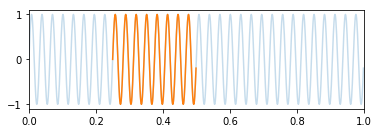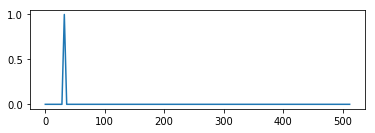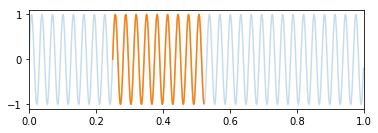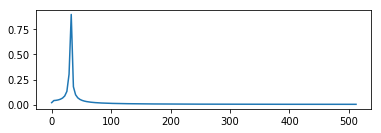### 增加频域分辨率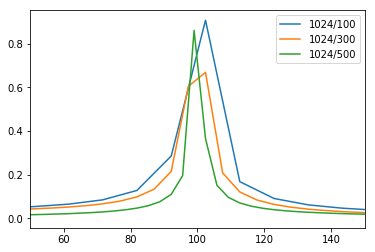### 窗函数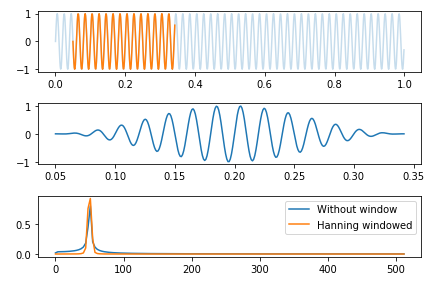### 平均

Perter Avitabile提到平均可以减少泄露。

### 参考文献

 Perter Avitabile. Modal Testing: A Parctitioner’s Guide[M]. Wiley. 2017